Courses

# Electronic Devices 5

## 10 Questions MCQ Test RRB JE for Electronics & Communication Engineering | Electronic Devices 5

Description
This mock test of Electronic Devices 5 for Electronics and Communication Engineering (ECE) helps you for every Electronics and Communication Engineering (ECE) entrance exam. This contains 10 Multiple Choice Questions for Electronics and Communication Engineering (ECE) Electronic Devices 5 (mcq) to study with solutions a complete question bank. The solved questions answers in this Electronic Devices 5 quiz give you a good mix of easy questions and tough questions. Electronics and Communication Engineering (ECE) students definitely take this Electronic Devices 5 exercise for a better result in the exam. You can find other Electronic Devices 5 extra questions, long questions & short questions for Electronics and Communication Engineering (ECE) on EduRev as well by searching above.
QUESTION: 1

### The figure shows the VTC characteristics of an NMOS inverter with three varying resistive loads (R).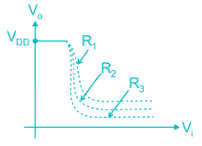The correct statement is

Solution: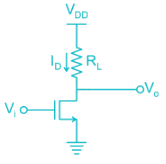As Vi is increased voltage drop across RL increases and V0 decreases.

The sharpness of the transition region increases with the increasing load resistance.

Hence R3 > R2 > R1.

*Answer can only contain numeric values
QUESTION: 2

### Assume that the zero for electrostatic potential is in the semiconductor bulk at large x and that there is no metal semiconductor work function difference. The relative dielectric constant for the oxide is ϵr = 11.8. If the intrinsic concentration is 1010/cm3. The doping density ND is _____ × 1017/cm3 (KT = 0.026 V)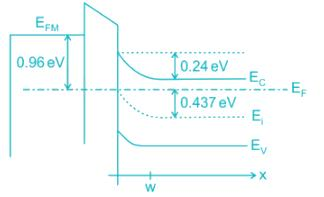Solution:

The doping density is given by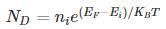= 1010 e(0.437/0.026)

= 1.99 × 1017

QUESTION: 3

### The figure shows MOS capacitor variation with applied gate voltage for n-type body/substrate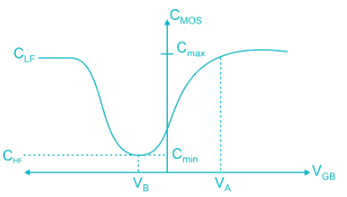The flat band voltage is

Solution: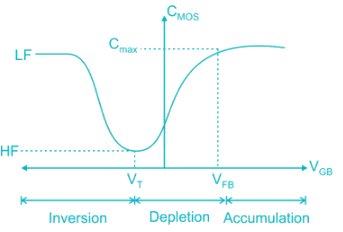Flat band voltage is the voltage where there is no charge present in oxide or oxide-semiconductor interface.

i.e. No thickness of channel

Cox is constant and decreased subsequently

Note: Here, VG is negative (for PMOS)

Hence, voltage is decreased from positive (VFB) to negative.

*Answer can only contain numeric values
QUESTION: 4

The insulator capacitance Ci of an ideal MOS capacitor with 10-nm gate oxide (εr = 3.9) on p-type Si with Na = 1016 cm-3 is ________ × 10-7 F/cm2

Solution:

The insulator capacitance is the capacitance under strong accumulation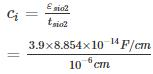= 3.453 × 10-7 F/cm2

QUESTION: 5

Which of the following curves represents the correct C – V characteristics of an NMOS transistor having an oxide layer thickness of 10 nm and a maximum depletion thickness of 100 nm. [Assume ϵs = permittivity of semiconductor, ϵox = permittivity of oxide and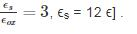Solution:

Given,

tox = 10 nm

d = 100 nm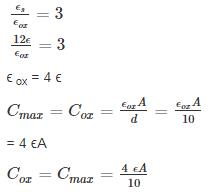Cmin = series combination of Cox and Cdep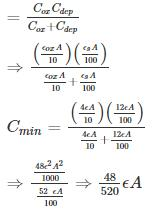= 0.09 ϵA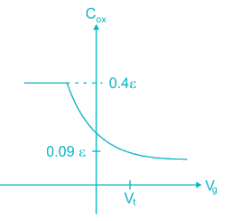QUESTION: 6

The mobility of hole is 0.4 times the mobility of electron. What must be the ratio of width of n-channel to p-channel MOSFET if they are to have equal drain currents when operated in saturation mode with same magnitude of overdrive voltage:

Solution:

ID-n = ID-p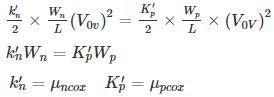μn Wn = μp Wp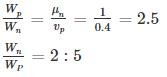QUESTION: 7

The circuit shown uses an NMOS transistor to implement a current source. For the transistor VTN = 1V and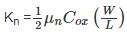=12.5 uA/V2. The required value of VGS to get IDC = 25 μA and corresponding compliance voltage is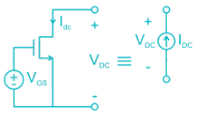Solution: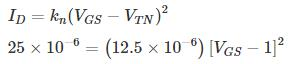2 = (VGS - 1)2‑

VGS = √2 + 1 = 2.414 V

To function as a current source, the transistor must be in saturation or

VDS > VDS (sat).

VDS (sat) = VGS - VTN

= 2.414 – 1

= 1.414.

Hence compliance voltage = 1.414 V.

Compliance voltage is the minimum voltage required to achieve the desired performance.

*Answer can only contain numeric values
QUESTION: 8

The output resistance R0 of the NMOS circuit if ID = 0.5 mA, λ = 0.02 V-1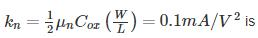_____ kilo ohms.Solution:

The small signal model with a test voltage Vx is shown.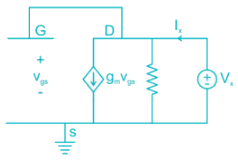The output resistance is given by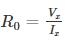From the circuit

Vgs = Vx

Applying KCL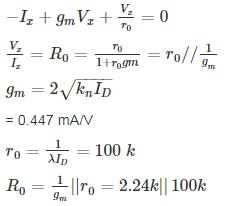QUESTION: 9

For a MOSFET with gate plate area 0.5 × 10-2 cm2 and oxide layer thickness 80 nm, the value of MOS capacitance and its break down voltage are, (assume relative di-electric constant of sio2, ϵr = 4 and ϵ0 = 8.854 × 10-14 F/cm and dielectric strength of sio2 film is 5 × 106 V/cm)

Solution:

Given gate plate area, which is also MOS capacitor area A = 0.5 × 10-2cm2

Oxide layer thickness tox = 80 nm

The value of MOS capacitance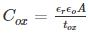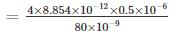= 0.22 μF

Dielectric breakdown happens of field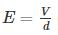greater than dielectric strength (5 × 106 V/cm)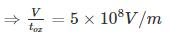V = 5 × 108 × 80 × 10-9 V = 40V

*Answer can only contain numeric values
QUESTION: 10

In the circuit shown in Figure, Transistors are characterized by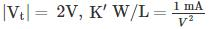and λ = 0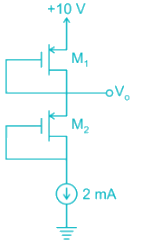The output voltage V0 is _______V

Solution:

Gate is connected to drain Both Transistor are in Saturation

For M1 Transistor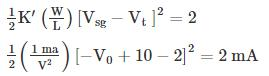⇒ (V - 8)2 = 4

V - 8 = ± 2

Taking +ve

V = 8 + 2 = 10 V (Not Possible)

Taking -ve

V = 8 - 2 = 6 V